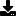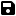Don't have an account yet? Register

# Function Validates IP address returns boolean

by Chris Morton (5 Submissions)
Category: String Manipulation
Compatability: VB.NET
Difficulty: Unknown Difficulty
Originally Published: Fri 10th June 2005
Date Added: Mon 8th February 2021
Rating:(1 Votes)

Function Validates IP address returns boolean

Rate Function Validates IP address returns boolean

`        Dim count As Byte        Dim dotcount As Byte        'check for illegal charaters        For count = 1 To Len(IPAddress)            If InStr("1234567890.", LCase(Mid(IPAddress, count, 1))) > 0 Then            Else                MsgBox("There are illegal characters")                Return False            End If        Next        'check if first character is "."        If InStr(IPAddress, ".") = 1 Then            MsgBox("First Character is '.'")            Return False        End If        'check if there are consecutive ".."        If InStr(IPAddress, "..") > 0 Then            MsgBox("There are consecutive '.'")            Return False        End If        'check for number of dots        For count = 1 To Len(IPAddress)            If Mid(IPAddress, count, 1) = "." Then                dotcount += 1                If dotcount > 3 Then                    MsgBox("There are two many '.'")                    Return False                End If            End If        Next        'check for values of ip address components         Dim num() = Split(IPAddress, ".")        For count = 0 To 3            If (num(count)) > 255 Then                MsgBox("IP address is invalid")                Return False            End If            'checks if last split is = 255            If num(3) = 255 Then                MsgBox("IP address is invalid")                Return False            End If        Next        MsgBox("Valid IP address")        Return True        'if all of these things are true return true    End Function`Download this snippetAdd to My Saved Code